Like   Tweet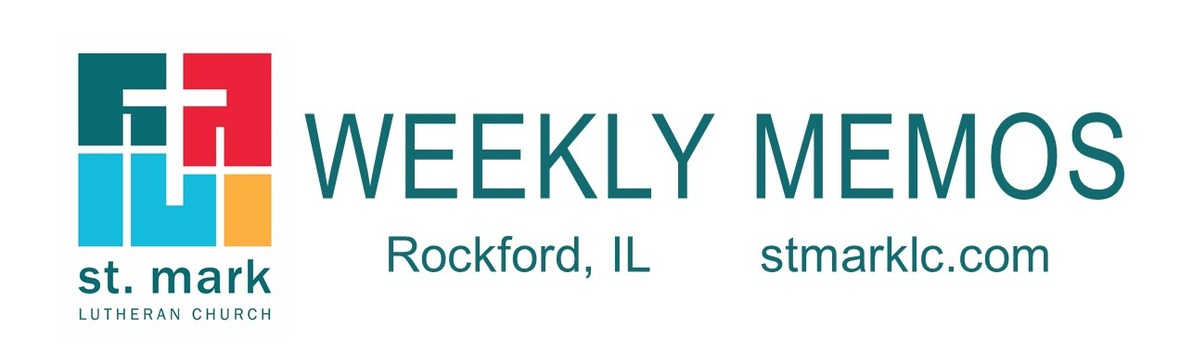## Pastoral Reflection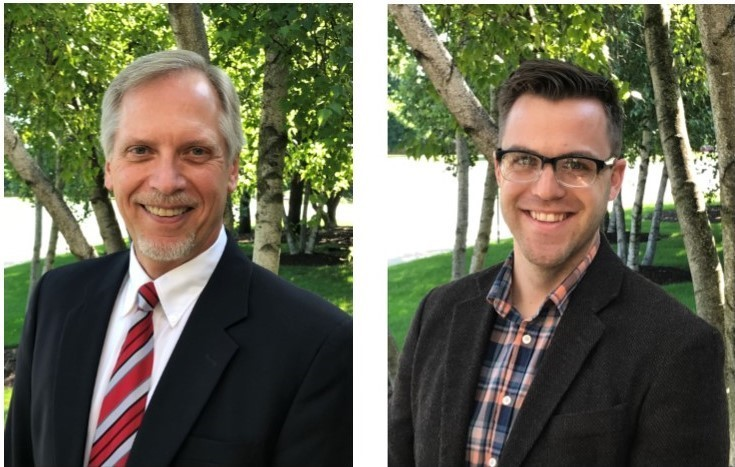Breaking News as We Begin 2020!

This month brings exciting changes at St. Mark. Read more.

 table div table+table+table+table div table{width:100%;padding:0}table div table+table+table+table div table img{width:96.23%;padding:0;float:none}table div table+table+table+table div table td{width:100%;padding:0 1.88% 18px}/* styles */## Sunday, January 12

8:00 A.M. Worship
9:30 A.M. Worship
9:30 A.M. Sunday School
9:30 A.M. First Communion Class
11:00 A.M. Worship
7:00 P.M. 2nd Sunday at Katie's Cup

## Monday, January 13

4:00 P.M. Deadline for MEMOS articles to be submitted
4:30 P.M. Yoga in the Cafe
5:00 P.M. Children's Ministry
6:30 P.M. Emotions Anonymous
7:00 P.M. Theology Pub

## Tuesday, January 14

10:00 A.M. Staff Meeting
11:00 A.M. St. Mark Duplicate Bridge
6:30 P.M. Worship & Music Ministry

## Wednesday, January 15

9:00 A.M. Women's Bible Study
9:00 A.M. Men's Bible Study
9:30 A.M. St. Mark Ringers
5:30 P.M. Good News Bearers
6:00 P.M. Bible Study
6:30 P.M. Confirmation
7:00 P.M. Voices of St. Mark

## Thursday, January 16

7:30 A.M. Panera Book Study
9:00 A.M. Cherry Valley Bridge Group
2:00 P.M. Barnabas Ministry
6:00 P.M. Diakonia
6:30 P.M. Emotions Anonymous
6:30 P.M. Blue Lights
7:00 P.M. Praise Band Rehearses

## Friday, January 17

7:30 A.M. St. Mark Men's Breakfast at Denny's

## Sunday, January 19

8:00 A.M. Worship
9:30 A.M. Worship
9:30 A.M. Sunday School
11:00 A.M. Worship

 table div table+table+table+table+table+table+table+table div table{width:100%;padding:0}table div table+table+table+table+table+table+table+table div table img{width:96.23%;padding:0;float:none}table div table+table+table+table+table+table+table+table div table td{width:100%;padding:0 1.88% 18px}/* styles */## News and Events

 table div table+table+table+table+table+table+table+table+table+table div table{width:100%;padding:0}table div table+table+table+table+table+table+table+table+table+table div table img{width:96.23%;padding:0;float:none}table div table+table+table+table+table+table+table+table+table+table div table td{width:100%;padding:0 1.88% 18px}/* styles */First Communion Class
The class is for first graders and older students who have not yet received their First Holy Communion. It will be held in a class room downstairs on January 12 during the Sunday school hour of 9:30-10:30 A.M. Pastor Chad
will instruct the class. Students will receive their First Holy Communion on Sunday, January 26 during the 9:30 A.M. service.

 table div table+table+table+table+table+table+table+table+table+table+table+table div table{width:100%;padding:0}table div table+table+table+table+table+table+table+table+table+table+table+table div table img{width:96.23%;padding:0;float:none}table div table+table+table+table+table+table+table+table+table+table+table+table div table td{width:100%;padding:0 1.88% 18px}/* styles */Theology Pub
Join Pastor Chad for a pint and great conversation on Monday, January 13 at 7:00 P.M. at Carlyle Brewing Co. ( 215 E. State St.) Please notice this is a week earlier than our normal time to meet.

 table div table+table+table+table+table+table+table+table+table+table+table+table+table+table div table{width:100%;padding:0}table div table+table+table+table+table+table+table+table+table+table+table+table+table+table div table img{width:96.23%;padding:0;float:none}table div table+table+table+table+table+table+table+table+table+table+table+table+table+table div table td{width:100%;padding:0 1.88% 18px}/* styles */Family Snow Tubing to Cascade Mountain

We will meet at St. Mark at 9:00 A.M. or you may meet us at Cascade at 11:00. Lunch will be on your own, there are concessions available for purchase in the tubing area. We will plan to head back to Rockford around 2 pm - arriving at St. Mark by 4 pm.

Tubing costs \$15 per student/adult (under 6 years old is \$12).

Please sign up with Melinda or Ann James by January 19th. You must sign-up so we can be sure to have enough cars/drivers.

Portage, WI 53901

 table div table+table+table+table+table+table+table+table+table+table+table+table+table+table+table+table div table{width:100%;padding:0}table div table+table+table+table+table+table+table+table+table+table+table+table+table+table+table+table div table img{width:96.23%;padding:0;float:none}table div table+table+table+table+table+table+table+table+table+table+table+table+table+table+table+table div table td{width:100%;padding:0 1.88% 18px}/* styles */St. Mark Mobile App!
This is different than our church directory app.
Our mobile app is designed specifically for St. Mark.

It will improve our communication with our congregation and nurture our members. A mobile app is important in the digital age. Never wonder how to get involved at St. Mark.

You will use this app for everything! You will be able to sign up for events, stay connected to your ministry group, give, send prayer requests, have an updated calendar, learn how to get involved and so much for.

We encourage you to download this app. The St. Mark app will be such a viable asset for you. It's about getting involved and staying connected.

If you have any questions, please call the office at 815-398-3557 or email Jill at Jill@stmarklc.com.

 table div table+table+table+table+table+table+table+table+table+table+table+table+table+table+table+table+table+table div table{width:100%;padding:0}table div table+table+table+table+table+table+table+table+table+table+table+table+table+table+table+table+table+table div table img{width:96.23%;padding:0;float:none}table div table+table+table+table+table+table+table+table+table+table+table+table+table+table+table+table+table+table div table td{width:100%;padding:0 1.88% 18px}/* styles */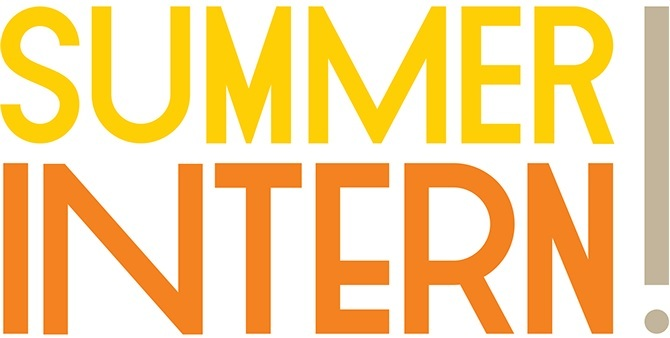Summer Intern Applications Now Available!
Every summer, our intern takes a central leadership role in Vacation Bible School and plans events for children, youth, and college-aged adults. This year our intern will also aid in leading the youth group on their mission trip. This 10-week position is a unique opportunity for college-age members of our congregation. Applications will be accepted from any St. Mark member under the age of 23 who graduated from high school in 2019 or earlier. The application deadline is February 15, 2020.
Appy Now.

 table div table+table+table+table+table+table+table+table+table+table+table+table+table+table+table+table+table+table+table+table div table{width:100%;padding:0}table div table+table+table+table+table+table+table+table+table+table+table+table+table+table+table+table+table+table+table+table div table img{width:96.23%;padding:0;float:none}table div table+table+table+table+table+table+table+table+table+table+table+table+table+table+table+table+table+table+table+table div table td{width:100%;padding:0 1.88% 18px}/* styles */The 2020 altar flower chart is up in Fellowship Hall on the cabinets next to the coffee station on Sunday mornings. The arrangements will be \$35. This is a great way to honor or remember that special
someone or that special anniversary.

 table div table+table+table+table+table+table+table+table+table+table+table+table+table+table+table+table+table+table+table+table+table+table div table{width:100%;padding:0}table div table+table+table+table+table+table+table+table+table+table+table+table+table+table+table+table+table+table+table+table+table+table div table img{width:96.23%;padding:0;float:none}table div table+table+table+table+table+table+table+table+table+table+table+table+table+table+table+table+table+table+table+table+table+table div table td{width:100%;padding:0 1.88% 18px}/* styles */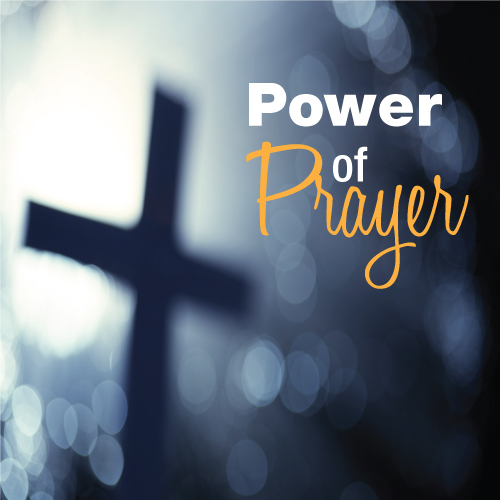St. Mark Prayer Chain
Would you like to be a part of the St. Mark prayer chain? Prayer requests come into the church office weekly. As we receive prayer requests we would send out an e-mail and ask that you pray daily for the persons mentioned in the requests. All requests are to remain confidential. Please e-mail melinda@stmarklc.com if you would like to participate in the prayer chain. “Be anxious for nothing, but in everything by prayer and supplication, with thanksgiving, let your requests be made known to God.” Colossians 4:2.

 table div table+table+table+table+table+table+table+table+table+table+table+table+table+table+table+table+table+table+table+table+table+table+table+table div table{width:100%;padding:0}table div table+table+table+table+table+table+table+table+table+table+table+table+table+table+table+table+table+table+table+table+table+table+table+table div table img{width:96.23%;padding:0;float:none}table div table+table+table+table+table+table+table+table+table+table+table+table+table+table+table+table+table+table+table+table+table+table+table+table div table td{width:100%;padding:0 1.88% 18px}/* styles */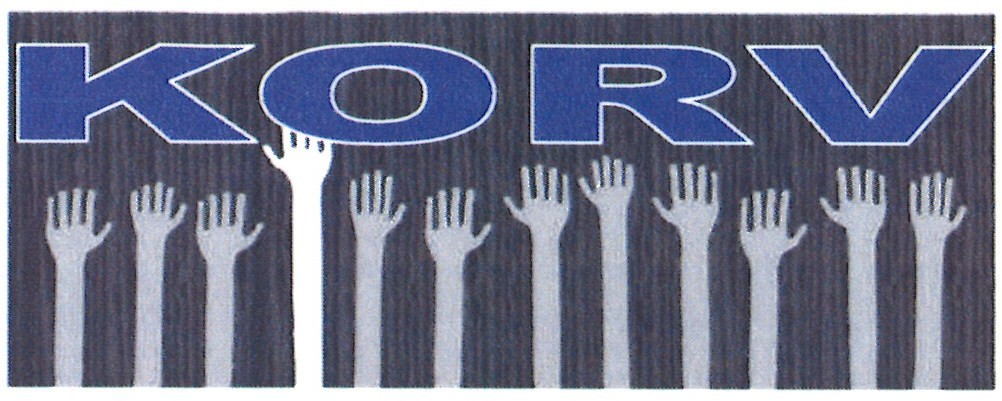Swedish Korv For Sale

 table div table+table+table+table+table+table+table+table+table+table+table+table+table+table+table+table+table+table+table+table+table+table+table+table+table+table div table{width:100%;padding:0}table div table+table+table+table+table+table+table+table+table+table+table+table+table+table+table+table+table+table+table+table+table+table+table+table+table+table div table img{width:96.23%;padding:0;float:none}table div table+table+table+table+table+table+table+table+table+table+table+table+table+table+table+table+table+table+table+table+table+table+table+table+table+table div table td{width:100%;padding:0 1.88% 18px}/* styles */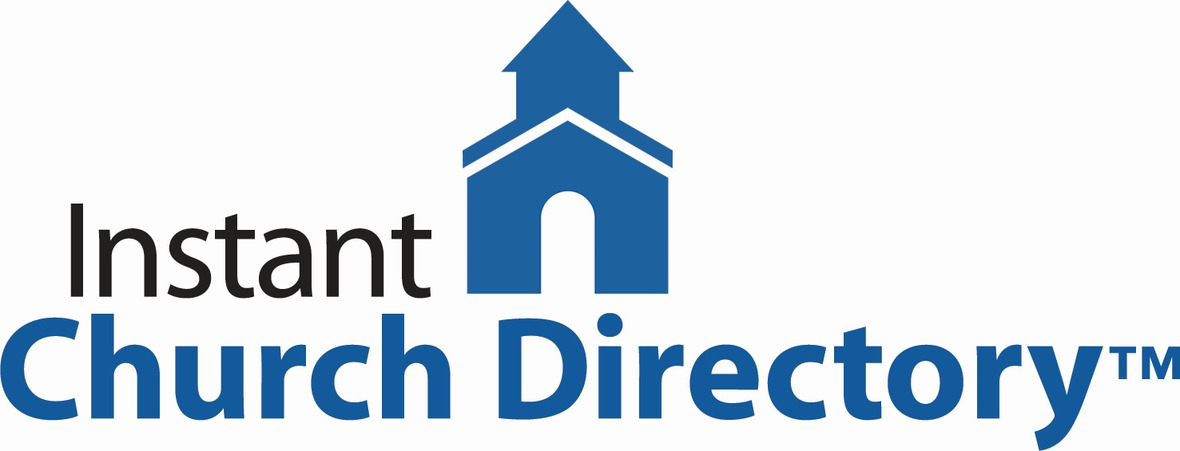Instant Church Directory
If you have not submitted a family photo for the online directory, please follow the links below.
It is simple and takes only a few minutes to upload your photo and then download the Instant Church Directory App. You will always have updated members' information at your fingertips.

Instant Church Directory

Once you have downloaded the app and created an account, you are not able to change or update your information, the system administrator is the only one to change and update the information. If something is incorrect, please contact the church office at 815-398-3557 or email jill@stmarklc.com.

Mobile App Login Instructions for Android & Kindle Devices

We also have a form for you to complete to make sure we have the most current information for the directory. There are paper forms at church or click on the link to Church Directory Form

 table div table+table+table+table+table+table+table+table+table+table+table+table+table+table+table+table+table+table+table+table+table+table+table+table+table+table+table+table div table{width:100%;padding:0}table div table+table+table+table+table+table+table+table+table+table+table+table+table+table+table+table+table+table+table+table+table+table+table+table+table+table+table+table div table img{width:96.23%;padding:0;float:none}table div table+table+table+table+table+table+table+table+table+table+table+table+table+table+table+table+table+table+table+table+table+table+table+table+table+table+table+table div table td{width:100%;padding:0 1.88% 18px}/* styles */We have been contacted by WeLearnUSA if we could announce that they are in desperate need of host families for Middle School Chinese students for 4 nights and 3 days. Jan 31-Feb 4. The students will visit Spectrum School and Rockford University. Please contact Maggie Kasicki at WeLearnUSA@gmail.com or 815-703-1133.

The students stay with their families for the weekend. They are here to experience a typical American household. The students are expecting to see piano lessons and all-day soccer games. That is real culture.

 table div table+table+table+table+table+table+table+table+table+table+table+table+table+table+table+table+table+table+table+table+table+table+table+table+table+table+table+table+table+table div table{width:100%;padding:0}table div table+table+table+table+table+table+table+table+table+table+table+table+table+table+table+table+table+table+table+table+table+table+table+table+table+table+table+table+table+table div table img{width:96.23%;padding:0;float:none}table div table+table+table+table+table+table+table+table+table+table+table+table+table+table+table+table+table+table+table+table+table+table+table+table+table+table+table+table+table+table div table td{width:100%;padding:0 1.88% 18px}/* styles */## Youth Ministry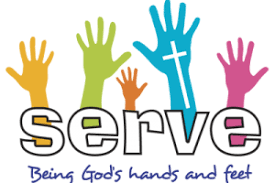Service Learning Camps, summer mission trip 2020 sign-up is happening!
If you are in 8th grade now through high school you are encouraged to join us. We will be traveling to Toledo, Ohio July 12-17th, 2020. The cost is \$350, with \$50 of that due now to secure a spot on the trip. See Melinda Alekna for more details. You may also email melinda@stmarklc.com or call/text 815-871-0390.
www.servicelearningcamps.com

SAVE the DATE
Saturday, March 21, Feed My Starving Children meal-packing in Schaumburg, Il.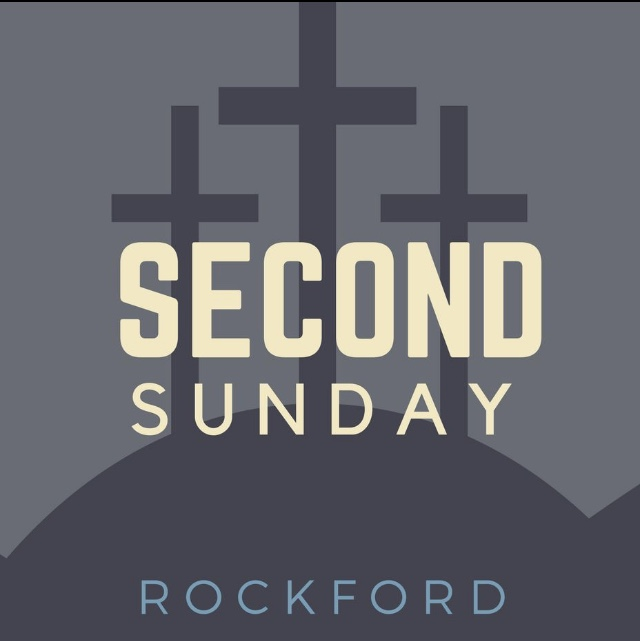Second Sunday is this Sunday, January 12, from 7:00-8:30 P.M. High School Youth and friends are welcome. Need a ride or more information? See Melinda Alekna or text/call 815-871-0390.

 table div table+table+table+table+table+table+table+table+table+table+table+table+table+table+table+table+table+table+table+table+table+table+table+table+table+table+table+table+table+table+table+table+table+table div table{width:100%;padding:0}table div table+table+table+table+table+table+table+table+table+table+table+table+table+table+table+table+table+table+table+table+table+table+table+table+table+table+table+table+table+table+table+table+table+table div table img{width:96.23%;padding:0;float:none}table div table+table+table+table+table+table+table+table+table+table+table+table+table+table+table+table+table+table+table+table+table+table+table+table+table+table+table+table+table+table+table+table+table+table div table td{width:100%;padding:0 1.88% 18px}/* styles */## Outreach Ministry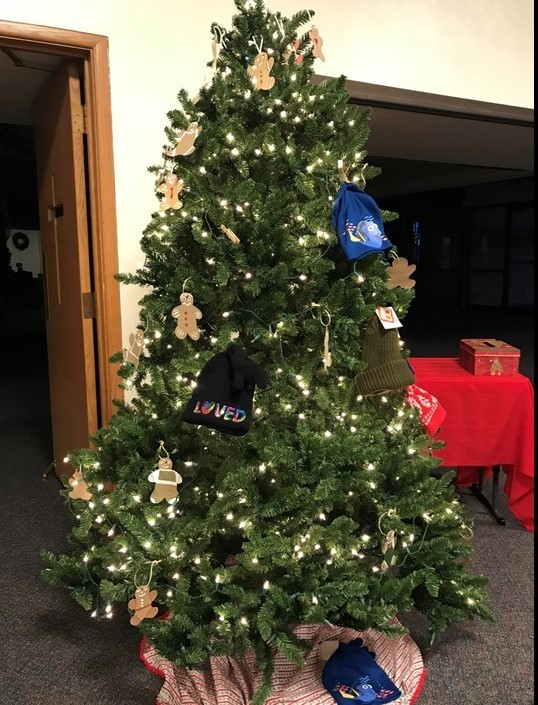Thank You!
Once again our St. Mark members have responded with open hearts by purchasing winter items for the special needs people served by Mosaic. The tree was full of hats, gloves, scarves, and mittens and there were even more items under the tree. Bags and bags of your gifts were taken to Mosaic at their office on Alpine Road and were gratefully received. The Outreach Committee thanks you for your great spirit and your generosity once again this year.

 table div table+table+table+table+table+table+table+table+table+table+table+table+table+table+table+table+table+table+table+table+table+table+table+table+table+table+table+table+table+table+table+table+table+table+table+table+table div table{width:100%;padding:0}table div table+table+table+table+table+table+table+table+table+table+table+table+table+table+table+table+table+table+table+table+table+table+table+table+table+table+table+table+table+table+table+table+table+table+table+table+table div table img{width:96.23%;padding:0;float:none}table div table+table+table+table+table+table+table+table+table+table+table+table+table+table+table+table+table+table+table+table+table+table+table+table+table+table+table+table+table+table+table+table+table+table+table+table+table div table td{width:100%;padding:0 1.88% 18px}/* styles */## Primetimers MinistryJanuary 22 WEDNESDAY - 12:30 - Our annual Potluck luncheon in the Common Ground Cafe. Sign up sheet available to post your special dish to pass. This is an opportunity for St. Mark members to start the new year by joining the Primetimers Ministry, meet the group and attend our monthly activities. Everyone is most welcome.

## Barnabas Ministry

 table div table+table+table+table+table+table+table+table+table+table+table+table+table+table+table+table+table+table+table+table+table+table+table+table+table+table+table+table+table+table+table+table+table+table+table+table+table+table+table+table+table div table{width:100%;padding:0}table div table+table+table+table+table+table+table+table+table+table+table+table+table+table+table+table+table+table+table+table+table+table+table+table+table+table+table+table+table+table+table+table+table+table+table+table+table+table+table+table+table div table img{width:96.23%;padding:0;float:none}table div table+table+table+table+table+table+table+table+table+table+table+table+table+table+table+table+table+table+table+table+table+table+table+table+table+table+table+table+table+table+table+table+table+table+table+table+table+table+table+table+table div table td{width:100%;padding:0 1.88% 18px}/* styles *//* styles */ Our next Barnabas meeting is on Thursday, January 16, 2020, in Common Grounds Café’.
 table div table+table+table+table+table+table+table+table+table+table+table+table+table+table+table+table+table+table+table+table+table+table+table+table+table+table+table+table+table+table+table+table+table+table+table+table+table+table+table+table+table+table+table div table{width:100%;padding:0}table div table+table+table+table+table+table+table+table+table+table+table+table+table+table+table+table+table+table+table+table+table+table+table+table+table+table+table+table+table+table+table+table+table+table+table+table+table+table+table+table+table+table+table div table img{width:96.23%;padding:0;float:none}table div table+table+table+table+table+table+table+table+table+table+table+table+table+table+table+table+table+table+table+table+table+table+table+table+table+table+table+table+table+table+table+table+table+table+table+table+table+table+table+table+table+table+table div table td{width:100%;padding:0 1.88% 18px}/* styles */## Our St. Mark members:

Tim Panjkovich, Carolyn Lee, Bette Patterson, Jason Carlson, Beverly Wright, Jim Tooley, Jerry Cazaniga, David Young, Keith Haggestad.

## Family and friends of St. Mark members:

Andrew Coffman and family, Pastor Jane McChesney, Sheryl Swanson, Emily Tropp, Louis Fivaz, Mike Leonard, Katelynn Audette, Eva Love Sherbondy, David Lundine, Clay Heinrich, Mary Graham, Kim Menke, Norma Comstock, Carolyn King, Ashton Henley, Nathan Gyllin.

## Those serving in the military and their families:

Tristan Davenport, Scot Hornick, Bailey Panjkovich, Xander Gehrke, Jim Tammen, Brandon Ballenger, Taylor Eversole, Dean Barron.

 table div table+table+table+table+table+table+table+table+table+table+table+table+table+table+table+table+table+table+table+table+table+table+table+table+table+table+table+table+table+table+table+table+table+table+table+table+table+table+table+table+table+table+table+table+table+table div table{width:100%;padding:0}table div table+table+table+table+table+table+table+table+table+table+table+table+table+table+table+table+table+table+table+table+table+table+table+table+table+table+table+table+table+table+table+table+table+table+table+table+table+table+table+table+table+table+table+table+table+table div table img{width:96.23%;padding:0;float:none}table div table+table+table+table+table+table+table+table+table+table+table+table+table+table+table+table+table+table+table+table+table+table+table+table+table+table+table+table+table+table+table+table+table+table+table+table+table+table+table+table+table+table+table+table+table+table div table td{width:100%;padding:0 1.88% 18px}/* styles *//* styles */ Office Hours Monday - Friday, 8:00 A.M. - 4:00 P.M. Telephone: 815-398-3557 Publications St. Mark Memos, the weekly e-newsletter, is distributed each Thursday. The deadline for submission is Monday by 4:00 P.M. Please click to send submissions. Sermons Online Miss a Sunday at St. Mark? Please visit our website and watch the sermons online. Please click to watch.